Home | | Maths 12th Std | Exercise 1.1: Inverse of a Non-Singular Square Matrix

# Exercise 1.1: Inverse of a Non-Singular Square Matrix

Mathematics : Applications of Matrices and Determinants: Inverse of a Non-Singular Square Matrix: Exercise Problem Questions with Answer, Solution (Maths Book back answers and solution for Exercise questions)

EXERCISE 1.1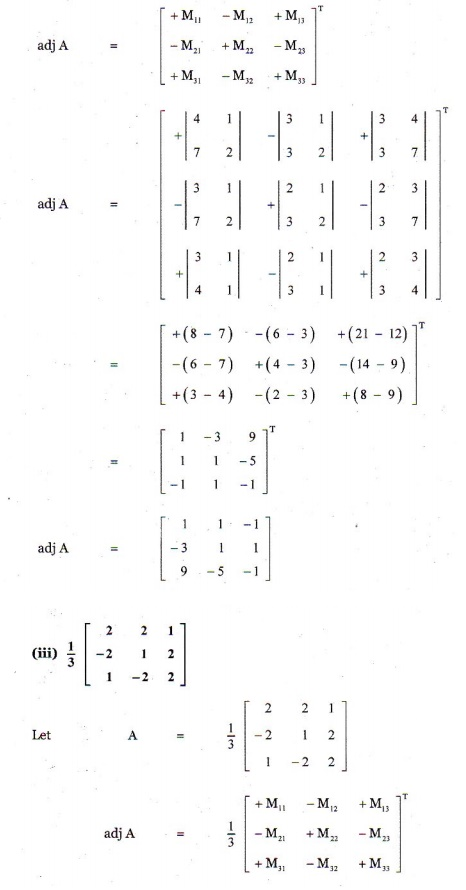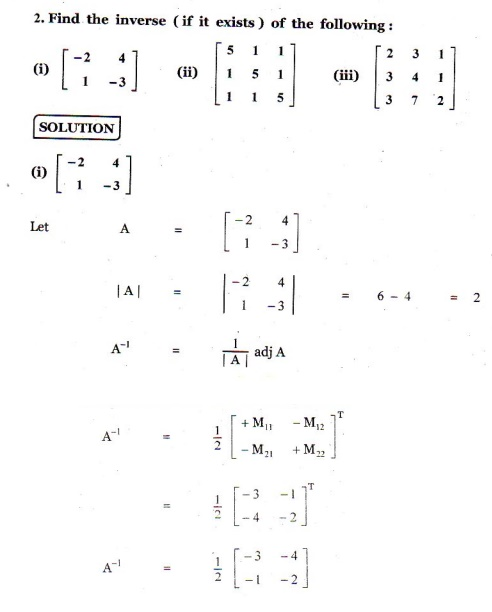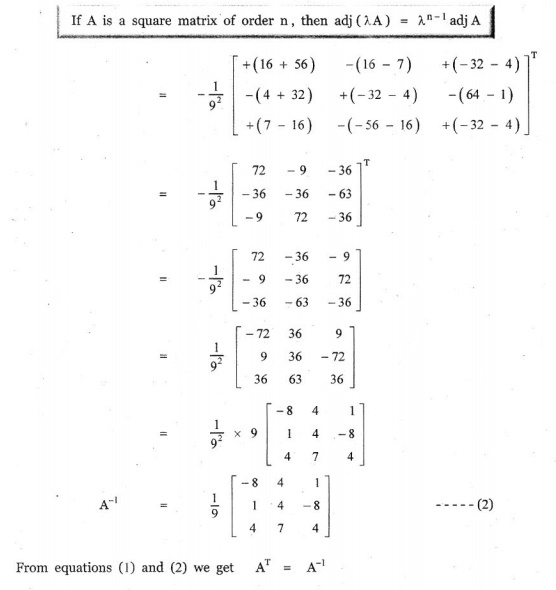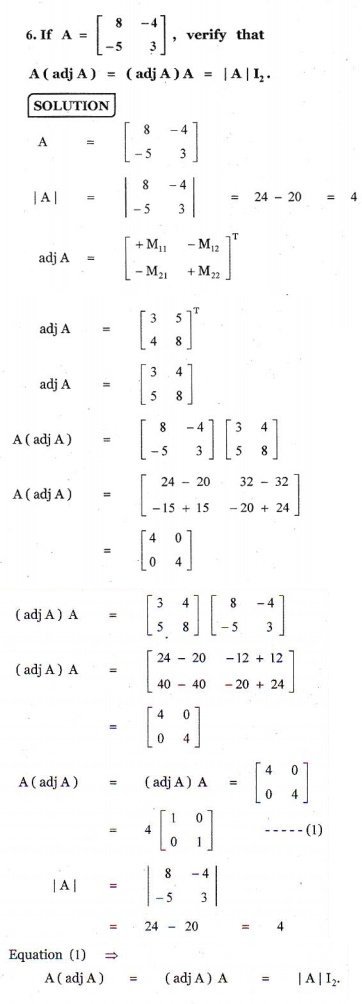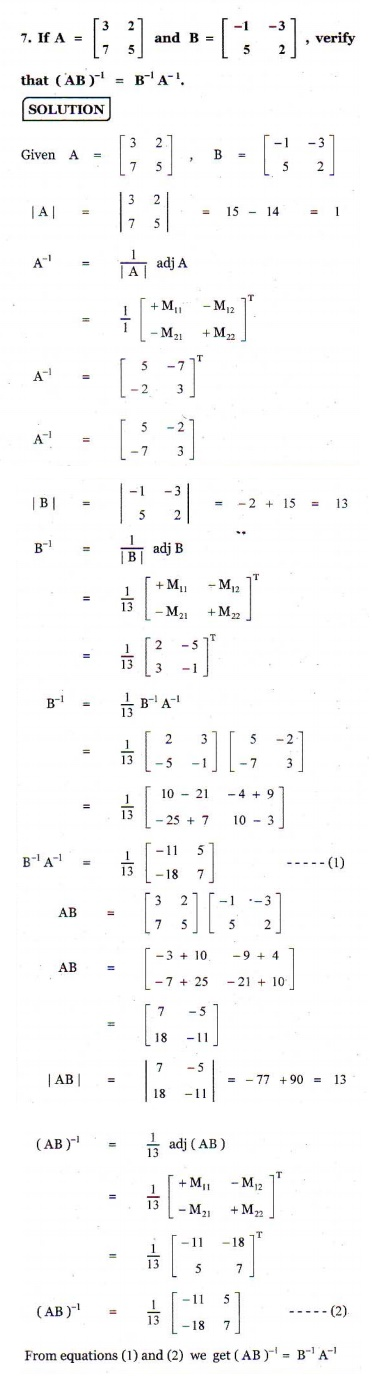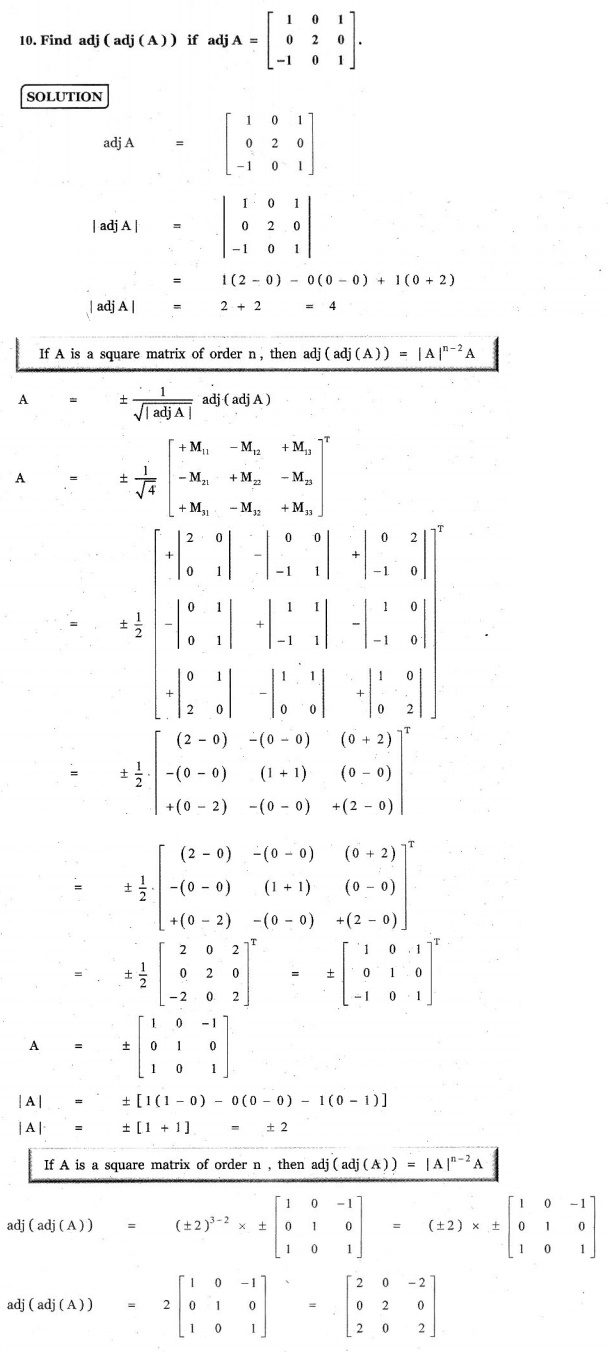EXERCISE 1.1

1. Find the adjoint of the following:2. Find the inverse (if it exists) of the following:15. Decrypt the received encoded message [2 ŌłÆ3] [20 4] with the encryption matrixand the decryption matrix as its inverse, where the system of codes are described by the numbers 1-26 to the letters A Z ŌłÆ respectively, and the number 0 to a blank space.